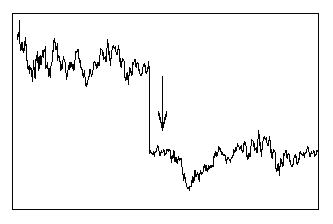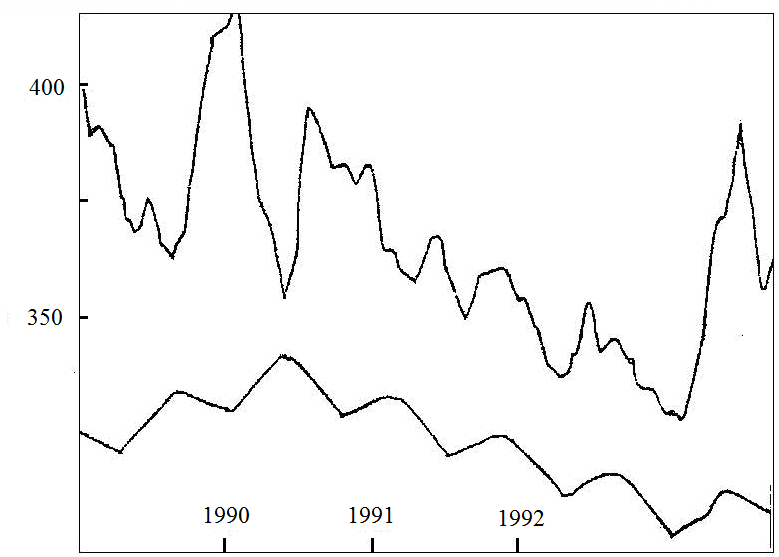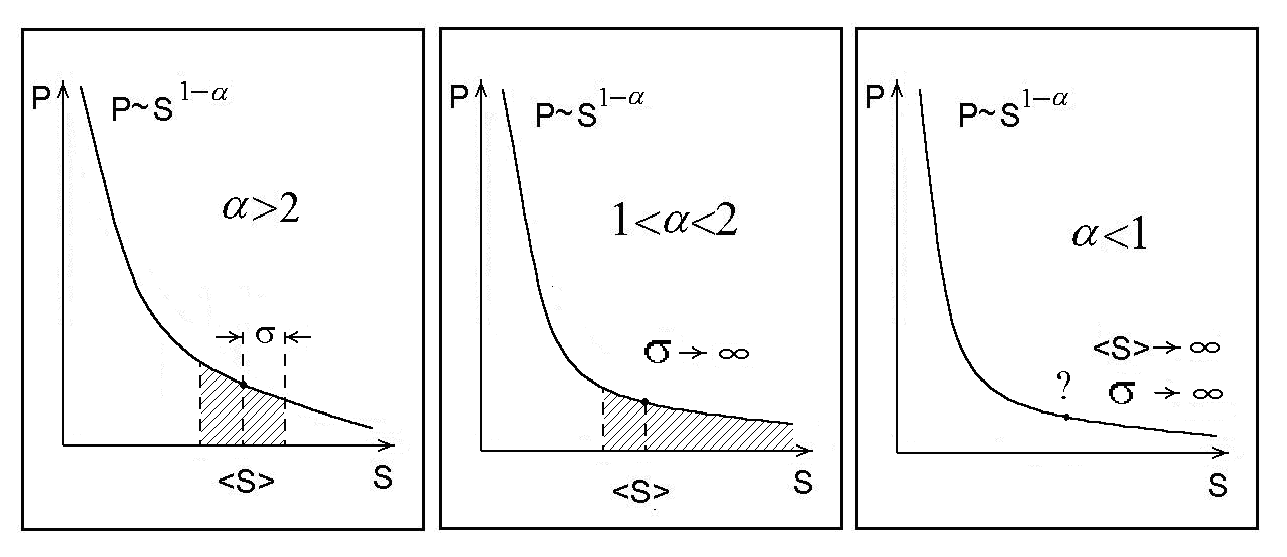# Economic Risk Theory (SPbSTU Press, 1999)

The modern approaches to forecasting critical conditions and catastrophic events in complex economic systems are introduced. Examples of application of quantitative methods of the economic risk theory to analysis of stability and diagnostics in economic systems based on statistical and/or nonlinear processing of time series are represented. The main attention is devoted to ways of holistic modeling of stochastic economic system behavior and problems of quantitative estimation of economic risks.

The textbook is designed for students of economic, marketing, management departments and for everyone who is interested in the problem of risk analysis in complex economic systems.

O. Y. Uritskaya. Introduction to Economic Risk Theory: Lecture Textbook // St. Petersburg: SPbSTU Press, 1999.

FULL TEXT REQUESTEnter the text above to confirm that you are a person:Fig. 1.  An example of bifurcation in model time series.Fig. 2.  Mont

hly fluctuations in world prices for gold (top) and the corresponding simulated cycle. (Cycles, 1993).Fig. 3. Dependence of the form of the Pareto distribution tail on the Pareto index value.

Contents

1. The subject of economic risk theory

1. Origin of risk in economic system

2. Characteristics of economic systems
3. Modeling of economic processes

4. Time series analysis

2. Basis of economic risk theory
1. Self-organized criticality theory

2. Self-organized criticality models
3. Fractals and their properties
4. Fractal objects

5. Fractal properties of economic systems

3. Methodology of economic risk theory
1. Economic risk estimation

2. Data preparation (measurements, aggregation, calendar problems)

3. Fractal dimension of time series (ruler method, box counting statistics, correlation function method, spectral method, Hurst rescaled range analysis)
4. Forecasting long-term tendencies on the base of time series fractal dimension

5. Analysis of system stability and catastrophe forecasting

6. Risk estimation by statistical methods

7. Risk estimation by fractal methods

4. Problems